Showing posts with label C programming. Show all posts
Showing posts with label C programming. Show all posts

## How to draw a line using c program

In this post i am going to tell how to draw a line using a c programming language.
The drawing can be performed in c program by including the header files <graphics.h>
here i am including my youtube video that will show you how to draw a line using the c program.

## How to find Factorial of a number using C program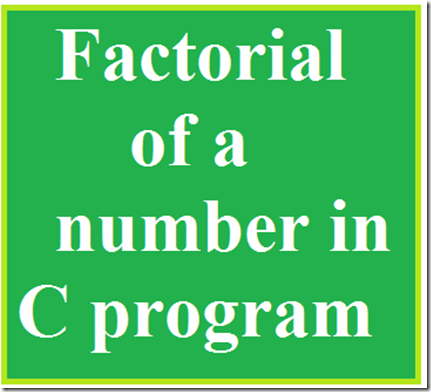Factorial of a number is nothing but the multiplies of every number in a specified number .
For example the factorial of the number 2 may be 1*2=2 , for 3 1*2*3=6.
It is easy to find the factorial of an number easily using c programming.
#include<stdio.h>
#include<conio.h>
Void main()
{
int i,n,fact;
clrscr();
printf(“\n Enter the number:”);
scanf(“%d”,&n);
for(i=1;i<=n;i++)
{
fact=fact*i;
}
printf(“\n the factorial of number %d is %d”,n,fact);
getch();
}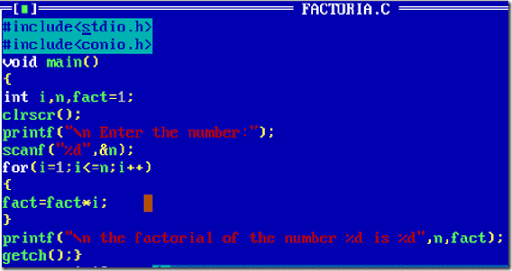Then after typing the above program in a compiler then compile it and run it and the output will be displayed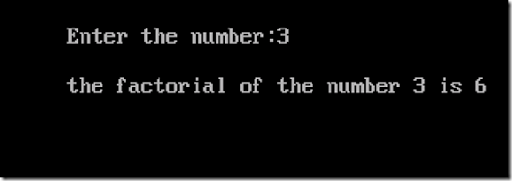Program Explanation:
In this program the first two lines represent the header files and then the void main() function from which the actual execution starts.
Then i have initialised three variables i , n and fact.
‘ n ‘ this variable i have used to get the input from the user, ‘ fact ’ is the variable used to store the factorial value that we needed and the variable ‘ i ’ is used to execute the for loop.
for(i=1;i<=n;i++)
{
fact=fact*i;
}
in this at first the fact=1 and i=1 is initialised  then the loop is repeated till the user input n .
at first time the loop executed
fact=fact*i that means
fact=1*1 so fact=1
then the i value is incremented and the condition is checked with the user’s input in this program the user’s input is 3.
So the loop is repeated till the i value becomes 3.
So for the next time the fact value will be
fact=2*1=2
and for the next time
fact=2*3=6
so the factorial of the number 3 is 6.
Thus we have simply calculated the factorial value of a number using c programming.
If you are confused in understanding the for loop concept then read this tutorial
Loop Controlled instructions.

## Learn C Language- Tutorial 5: Loop Control Instructions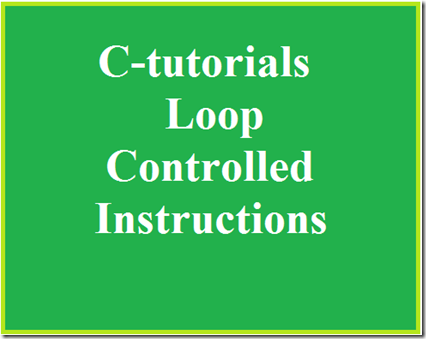So far we have seen the basics of C programming and how to get input from the users and some decision control instructions in the previous tutorials. In this tutorial we will learn about the use of Loop controlled instructions in detail.
Loops are used to execute certain statements repeatedly to until a certain condition is met. This involves repeating some portion of the program in either a specified number of times or until a particular condition is being satisfied.
This repetitive operation is done through a loop control instructions.
There are three methods by way of which we can repeat a part of a program . They are
a) Using a for statement
b) Using a while statement
c) Using a do-while statement

#### While Loop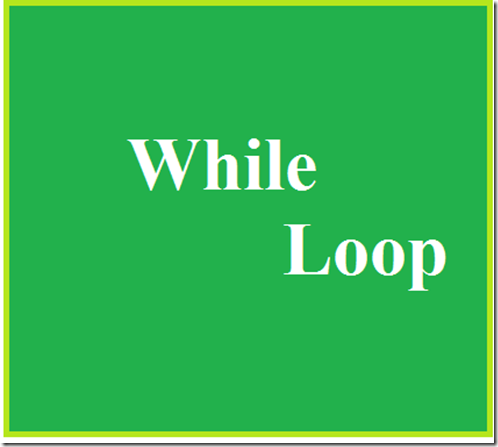It is often the case in programming that you want to do something a fixed number of times. For example getting marks of 5 subjects for 5 students to calculate total marks and generating Ranks among them, getting salaries of n employees and calculating their basic pay, da, etc...
For these purpose we will use this Loop control instructions.
The While loop can be used to solve above situations.
#include<stdio.h>
#include<conio.h>
void main()
{
int p,n,count;
float r,si;
count=1;
while(count<=3)
{
printf(“\n Enter values of p,n,r”);
scanf(“%d%d%f”,&p,&n,&r);
si=p*n*r/100;
printf(“Simple Interest =Rs.%\n f”,si);
count=count+1;
}
return 0;
}
In the above program uses while loop for calculating simple interest for 3 times .
We already know that the first two line of the program is header files , and the next line is the main function from where the actual program starts.
Then the initialization of variables, after that the while loop starts the general form of while loop is that
while(condition)
in this program the condition for while loop is count <=3
it is that the the variable first initialised to the value 1, inside the loop the operation for calculating the simple interest, inside the while condition , the condition is that when the count value incremented from 1 to 2 and 3 the operation must be continued to find the interest till it reaches 3 . Once the count value is reaches 3 the while loops execution stops and the output is displayed .
To increment the count value the line count=count+1
is given. Thus the output is obtained.

#### For Loop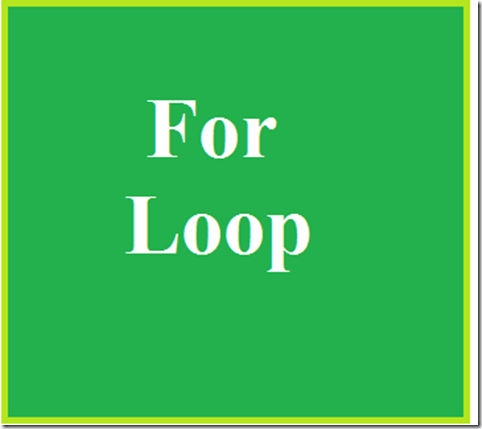In while loop the initialisation of the variable is outside the loop and it is must to check the condition of the logic , then that variable must be incremented , so that the loop is incremented and the condition is checked until it is satisfied.
It is a very long process, so that the programmers prefer for loop instead of while loop , because this for loop provides initialisation, condition checking and increment in a single line itself.
Syntax for For loop is as follows
for( initialisation ; condition; increment/decrement)
for example
for(i=0;i<=5;i++)
so it is very easy for the programmer to check certain condition.
Let us see an simple program that implements for loop
#include<stdio.h>
#include<conio.h>
void main()
{
int i,n;
clrscr();
printf(“\n Enter the total number :”);
scanf(“%d”,&n);
for(i=1;i<=n;i++)
{
printf(“\n The numbers are %d”,i);
}
getch();
}

Let we code this in an compiler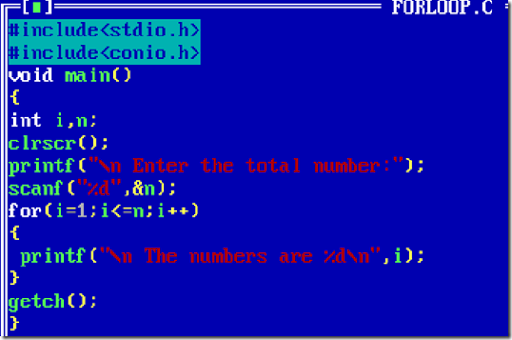Then compile it using Alt+F9 and run it by Ctrl+F9.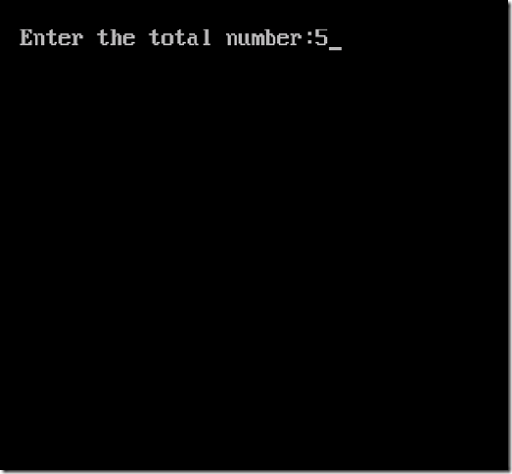provide some numbers then click enter
we get the output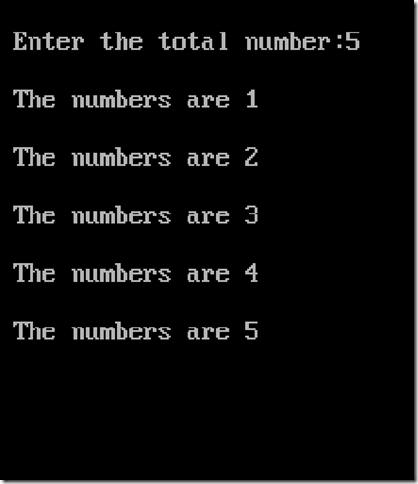Thus we got the output .
Program Explanation:
As we know that the first two lines of the program is including the header files.
Then as usual the void main function, in this program we simply going to get an input number from the user for example and print from 1 to that particular number using the for loop.
For this we need two variables i and n. i is for initialising the values to carry out the for loop and n for getting input from the user.
Then we start the for loop
for(i=1;i<=n;i++)
in this statement i=1 is assigned so that the loop starts from 1 then the i<=n is given so that the loop repeats until the value of i is less and equal to the n , the i++ is incrementing the value of i, so that the loop is executed iteratively.
So , i think you might now understood the importance of the for loop.

#### Do-while Loop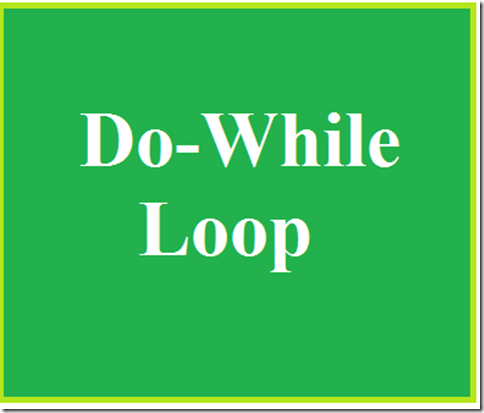There is a minor difference between the working of while and do-while loops. This difference is the place where the condition is tested.
The while  tests the condition before executing any of the statements within while loop.
In do-while tests the condition after having executed the statements within the loop.
The syntax is:
do
{
this;
and this;
and this;
….
}
while(this condition is true);
This means that do-while would execute its statements at least once, even if the condition fails for the first time.
For example to understand the difference between while and do-while consider the following example
#include<stdio.h>
#include<conio.h>
void main()
{
while(4<1)
printf(“Hello world”);
return 0;
}
In this above program the printf statement will not be executed since it does not satisfy the condition .
In case of do-while
#include<stdio.h>
#include<conio.h>
void main()
{
do
{
printf(“\n hello world”);
}while(4<1);
return 0;
}
In the above program the printf statement is executed at east once then only it checks the condition.
Thus the do-while loop can be understood easily.
I think this content is very useful and informative to all the new programmers.
Related  C tutorials:
Getting started with C
How to run c program
How to add two numbers in C
decision control instructions

## Learn C Language-Tutorial 4: Decision Control Instructions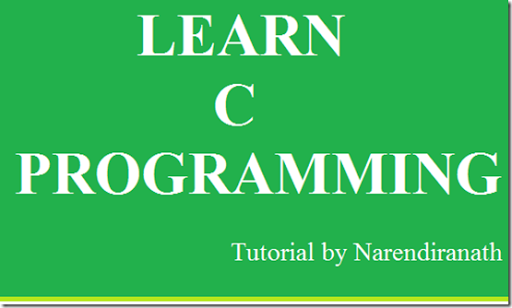In this tutorial we will learn about the Decision Control Instructions which makes a basics for any applications. In real time there are several conditions applied to complete a task.
In C programming we can implement the conditions by using these Decision Control Instructions.
They are
1.The if statement
2.The if-else statement
3.The conditional operator
Let we look in detail about these…
The if statement:
The if  is a keyword in  C language to implement the decision control instructions.
The general form of if statement is
if(this condition is true)
execute this statement;
The condition that we have to apply is written within the parenthesis.
Let us see an example
#include<stdio.h>
#include<conio.h>
void main()
{
int a;
clrscr();
printf(“\n Enter the number less than 10”);
scanf(“%d”,&a);
if(a<10)
printf(“you have entered corresct number”);
return 0;
}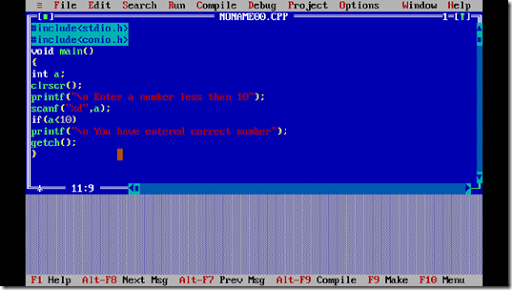Compile it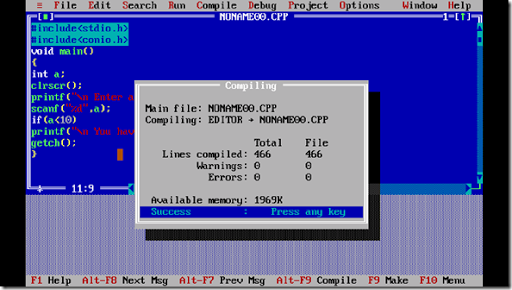Run it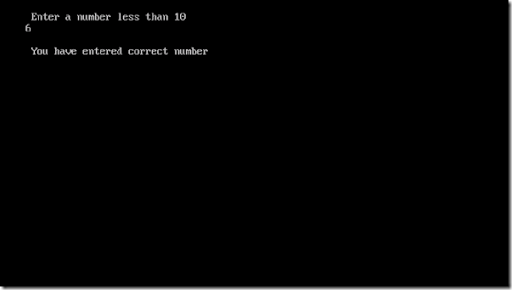if you enter the number greater than 10 then the output screen will be returned to the programming page.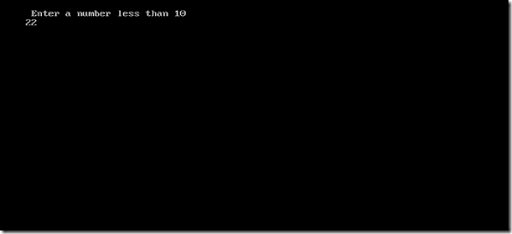In the above program if the wrong input is given the system will not show anything to the user it just change the output screen to the original screen.There is no notification that the users input is wrong thus an another conditional statement is used it is the if-else statement.
The if-else statement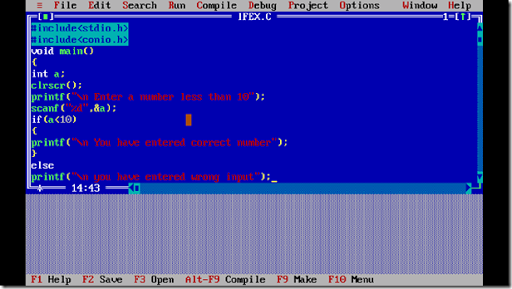#include<stdio.h>
#include<conio.h>
void main()
{
int a;
clrscr();
printf(“\n Enter the number less than 10“);
scanf(“%d”,&a);
if(a<10)
{
printf(“\n You have entered correct number”);
}
else
printf(“\n you have entered wrong number”);
getch();
}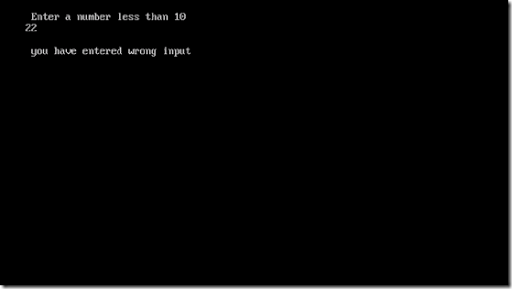Thus we are getting the output
Forms of IF
the if statement take the following forms
1. if(condition)
do this;
2.if(condition)
{
do this;
and this;
}
3.if(conditon)
do this;
else
do this;
4.if(condition)
{
do this;
and this;
}
else
{
do this;
and this;
}
5.if(condition)
do thus;
else
{
if(condition)
do this;
else
{
do this;
and this;
}
}
if(condition)
{
if(condition)
do this;
else
{
do this;
and this;
}
}
else
do this;

Use of Logical Operator

C language supports 3 logical operators 'AND' operator '&&','OR'operator ' ||' , and 'NOT' ! operators.
&& - this operator is to check the conditions and it will expect that every conditions associated with must be true otherwise it will not be executed.

||- The OR operator will be satisfied with any of its associated condition is true.

!- The NOT operator will perform not equal to operation.

Let us see an example to understand the above operators.

#include<stdio.h>
#include<conio.h>
void main()
{
int m1,m2,m3,m4,m5,tot,per;
printf("\n Enter the marks of 5 subjects");
scanf("%d%d%d%d%d",&m1,&m2,&m3,&m4,&m5);
tot=m1+m2+m3+m4+m5;
per=tot/5;
if(m1>=35&&m2>=35&&m3>=35m4>=35&&m5>=35)
{printf("\n pass");}
else
printf("\n Fail");
if(m1>85||m2>85||m3>85||m4>85||m5>85)
{
printf("\n eligible for distinction")
}
else
printf("\n Not elligible");
if(per!>85)
{
printf("\n not a rank holder");
}
else
printf("\nCongradulations... You are a  Rank Holder");
getch();
}

Program Explanation:

In the above program pass will be displayed only if all the marks are greater than or equal to 35.

The eligible for distinction if any one of the mark is greater than 85.

The Rank holder is decided if the percentage 'per' is not greater than 85.

I thing you have understood the Logical operator...

The Conditional Operator

The Conditional operators ? and : sometimes called as ternary operators since they take three arguments.

Their general form is

expression 1? expression 2:expression 3

that is  "if  expression 1 is true then the value returned will be expression 2 otherwise the value returned will be expression 3"

Let us see an example:

#include<stdio.h>
#include<conio.h>
void main()
{
int a,b;
clrscr();
printf("\n Enter the number");
scanf("%d",&a);
b=(a>10?5:6);
printf("\n the result is %d",b);
getch();
}

Thus in this program if the value of a is greater than 10 then the value of b is 5 otherwise the value is 6.

That's all guys we have done learning this tutorial of Decision Control Structure

read it , any doubt comment below if any doubt , share it ......

## Learn C Language–Tutorial 2: Getting started with C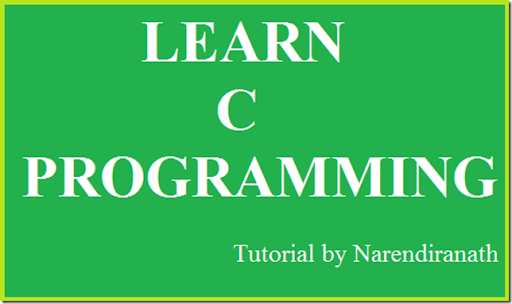Learn C Programming is a series of tutorials on c language. Follow my simple instructions and You also can become the C programmer.
In the first tutorial I have said how to run a C program. You can view the tutorial by clicking the link
http://www.millioninformations.com/2015/08/how-to-run-c-program.html
In that tutorial I have said about What is a C language and the method of how to run the C language. But I have not yet told about the basic things in the C program.
To be tell this in detail consider an example that we learn English language since it is a global language for communication. We do not learn English in a single day, first we learn the alphabets and then we use the alphabets to combine the letters and form a word. Then we learn some grammar to create a sentence. Refer the diagram for clear understanding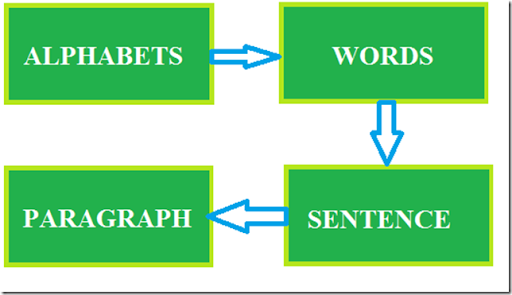Like that in order to learn a C language there are some basic things we need to learn like alphabets, numbers, keywords, special symbols, constants, variables and how these are constructed and used in the C program.
Steps involved in learning C language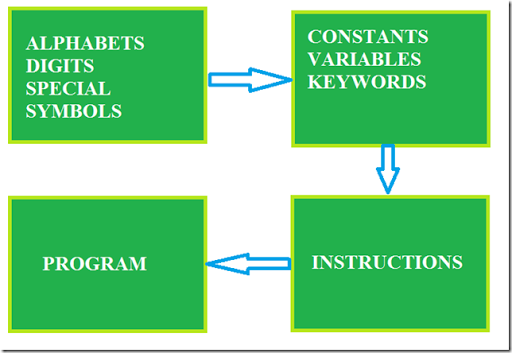In this tutorial i am going to mainly concentrate on the first boxAlphabets – A,B……Z and a,b….z
Digits – 0,1,2,3,4,5,6,7,8,9
special symbols – ~!@#\$%^&*()_+=-/?”’>.<,:;[]{}
The alphabets, numbers,and special symbols when properly combined form constants, variables and keywords.
Constants and Variables
A Variable is simple to describe let us assume that to perform a simple addition calculation manually for example
we simply write it in a paper as
5
+
3
----
8
----
So we need a space to write the numbers for calculation for manual calculations we use paper and pen.
So in case of computer calculation how do we do ???
So a storage is required in terms of storing the numbers for calculations. Thus we use alphabets to store the numbers.
we use lower case letters to store a number.
That alphabet is called as a variable and the number stored is called as constants.
In order to use a variable to store some values we need to say to the computer that i am going to use this particular letter to store a value this process is called as variable declaration.
Consider the above example
Consider the below is the storage in computer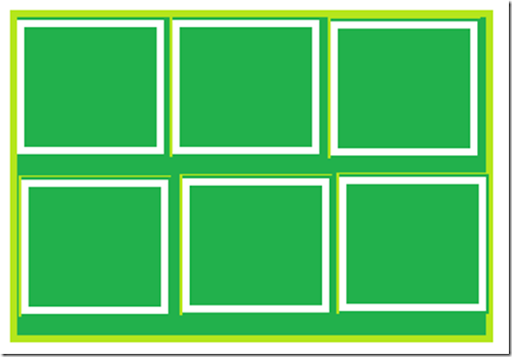If i am going to use a for storing 5 and b for storing 3
and c for storing result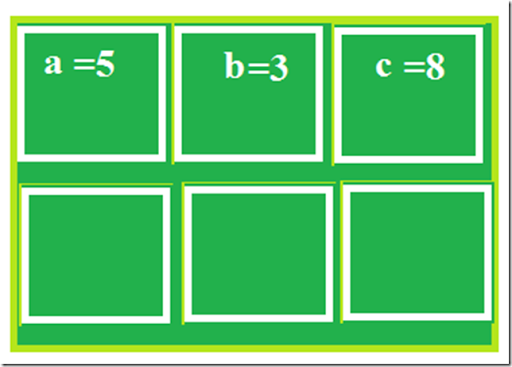Thus the concept of variables and constants are clearly explained.
TYPES OF C CONSTANTS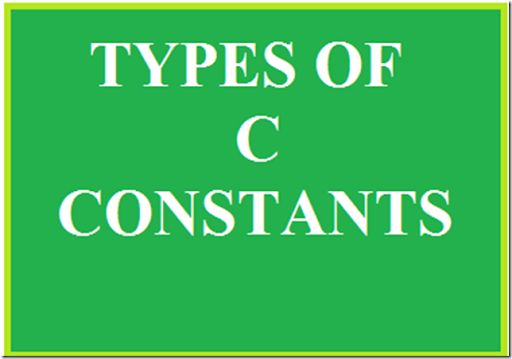There are two types of constants
1.Primary Constants
2.Secondary Constants
Primary Constants includes
• Integer Constants
• Real Constants
• Character Constants
Integer Constants
An Integer Constant must be at least of one digit, it can be a negative or positive the integer constant lies between the range –2147483648n to +2147483647.
ex: 1,2,3000,-250
Real Constant
These are also called as Floating point constants.
A real constant must have at least one digit, it must have a decimal point, default sign is positive but it could have both positive and negative.
ex: +445.566 , –56.45, 456.0
Character Constants
A character constant is a single alphabet written within a inverted commas.
ex: ‘A’ , ‘a’
Types of Variables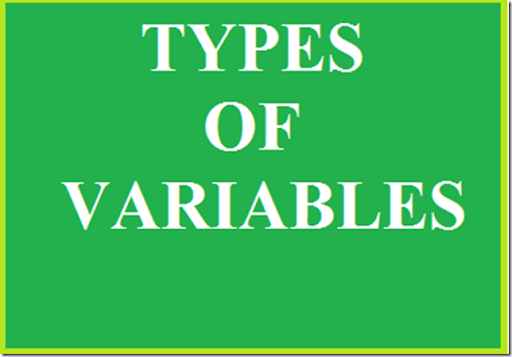The Variable is different for different data types. Data Type is nothing but it is a type of the data that it is a integer or character or String or float.
A variable names are names given to the storage location in memory.
A variable name is any combination of 1 to 31 alphabets, digits,underscores.
The first letter of the variable must be an alphabet or underscore.
No commas or blanks are allowed, no special symbols other than underscore should not be used.
ex: mat_marks, m5_marks, emp_name
If you notice that mat_marks and m5_marks are marks that are numbers and emp_name is a collection of character so how the complier will differentiate between these .
Here the data type is used
The numbers are int types names are char types
decimal values are float types.
ex: int num, char name, float percntage.
Now we are in the final discussion of this tutorial that it is Keyword.
C KEYWORDSKeywords are the predefined words whose meaning is already explained to the C compiler.
The Keywords cannot be used as a variable names.
There are 32 keywords available in C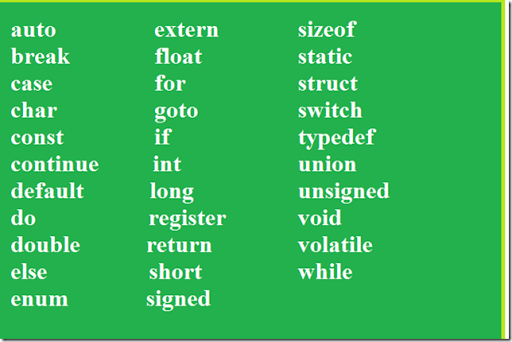In our upcoming tutorials we can deeply see about the above keywords.
I hope this tutorial will thought you some tips in c.
Keep visiting my blog …
stay tuned for further tutorials…

## Learn C language - Tutorial 3 : How to add two numbers in CAddition of number program is the basic program in C or any other language.
In the first C tutorial I gave the basic C code to display “This is my first C program”.
In this post i will give the tips to add numbers in C program.
There are two types of implementing this program.
In the first method We can add the numbers by declaring the values at the programming time itself this method is called as “Static method”.
Let we see how to add the numbers using this method.
Type the following code in the C compiler for example Turbo c++
#include<stdio.h>
#include<conio.h>
void main()
{
int a=5,b=3,c;
clrscr();
c=a+b;
getch();
}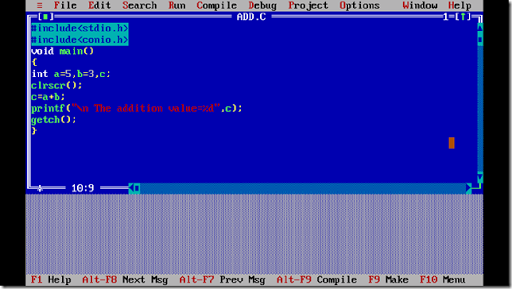After typing the code press F2 to save and save it as add.c the saving syntax for c is filename.c
Then we need to compile the program using ALT+F9.
Then run the program by pressing CTRL+F9
You can see the output below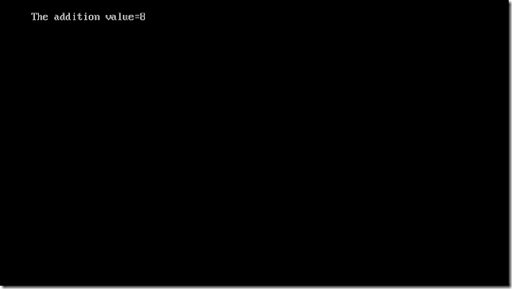You can see that the addition value of5 and 3 is 8.
PROGRAM EXPLANATION
The first two lines are called as Header Files
The first line of the program is
#include<stdio.h>
Stdio stands for Standard Input and Output ,
this is used to get the input from the show the output to the user by using predefined(already present in the C library) functions(it is nothing but it do the some task in this case input and output) for example: printf for printing the output in the screen, scanf for getting input from the user.
The second line is
#include<conio.h>
This is Console input and output it is used for connecting the consoles for example: clrscr() this is used to clear the screen of the monitor to erase the previous output of the program you have done before.
the next is
void main()
This is the main function from where the actual programming starts.
void is a return type which returns nothing but it mostly used in order to avoid some errors.
the next is
{
}
this denotes that the coding must be inside this braces.
The next line is
int a=5,b=3,c;
The above line is called as declaration statement(it means saying to the program that i am a part of this program with the certain values).
int is called as Data Type.
Data Type means the type of the data , in the case of addition program we use the data of integer (number) types thus we are using int data type , if we operate on names we use char data type (i.e character ).
Next the a,b,c are called as variables.
Variables are nothing but creating a storage space for the values to be stored, since we are storing multiple values we use multiple variables.
In the above program we used static method so we have declared the values for the variables.
The next line is clrscr() , this is a function that is used to clear the output screen.
The next line is
c=a+b;
The next line in the program is
printf() is the function that is used to print the output on the screen.
the output to be displayed is written within the double quotes “ ”
the %d is for printing the integer value and the c is for printing the addition value stored in the variable c.
the next line is
getch() function is used to print the output stable, if you are not putting this line the compiler will not show the error but the output will not be displayed thus this is very important.
If you notice at the end of each line i have put semi colon ; it indicates that end of the statement.
I hope you have understand the basic concepts in programming.
Let we go to the next method of addition is by getting the input of the two variables from the user
Type the code in the Turbo c++
#include<stdio.h>
#include<conio.h>
void main()
{
int a,b,c;
clrscr();
printf(“\n enter the value of a:”);
scanf(“%d”,&a);
printf(“\n enter the value of b:”);
scanf(“%d”,&b);
c=a+b;
getch();
}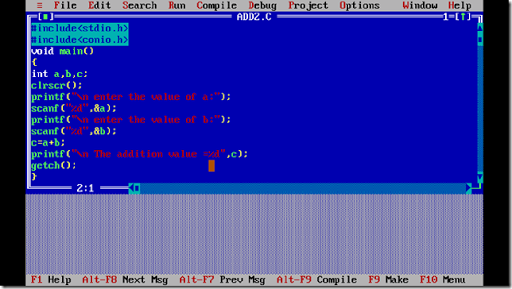Then compile the program and run the program
First it will ask for the value of a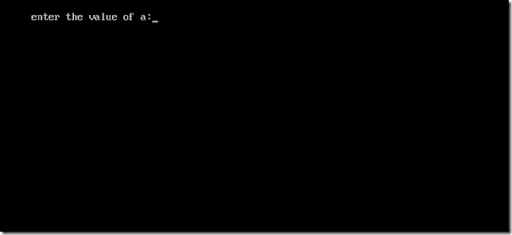Enter the value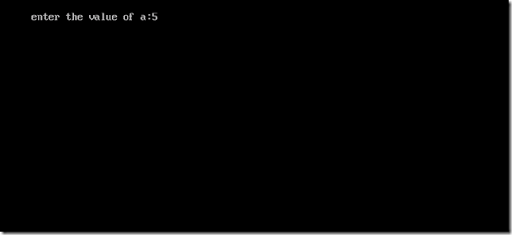Then it will ask for the value of b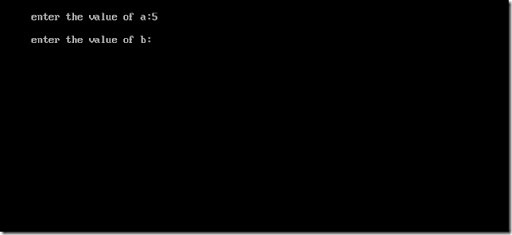Then give the value for the variable b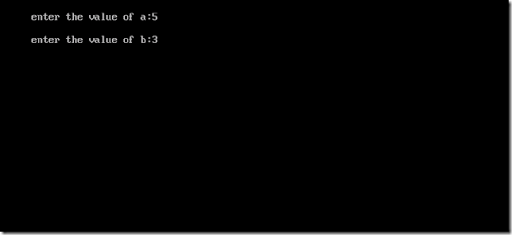Then the result will be displayed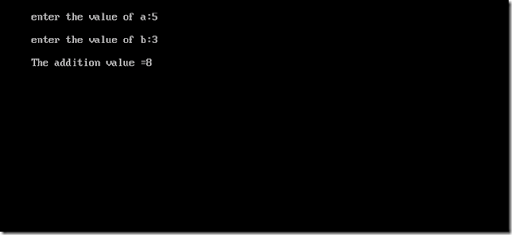That’s it we have written the program to add two numbers in two different ways…
Hope the tip was useful…

## How to run a c++ programHai, this tutorial will teach you how to run a c ++ program, although you don’t have much knowledge about it.
Let me something tell about C++. C++ is a middle-level programming language developed by Bjarne Stroustrup starting in 1979 at Bell Labs. C++ runs on a variety of platforms, such as Windows, Mac OS, and the various versions of UNIX.

In the early 1970s, Dennis Ritchie of Bell Laboratories was engaged in a project to develop a new operating system.  Ritchie discovered that in order to accomplish his task he needed the use of a programming language that was concise and that produced compact and speedy programs. This need led Ritchie to develop the programming language called C.

In the early 1980's, also at Bell Laboratories, another programming language was created which was based upon the C language.  This new language was developed by Bjarne Stroustrup and was called C++.Developed at AT&T bell laboratoryThe C++ program is written in high level language and it cannot be directly understand by the system, so a compiler(converts high level language to machine level language) is needed.
I prefer Turbo c++ compiler in which we can compile both c and c++ programs.Let we start our coding…..
In order to program we need a compiler so download Turbo c++ software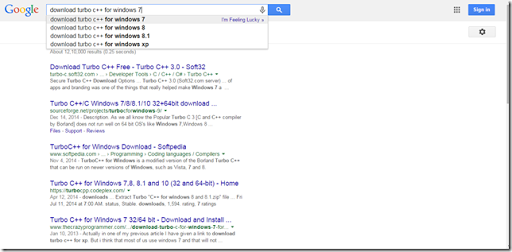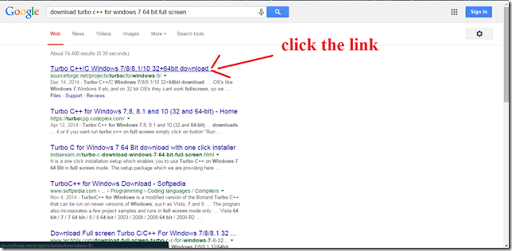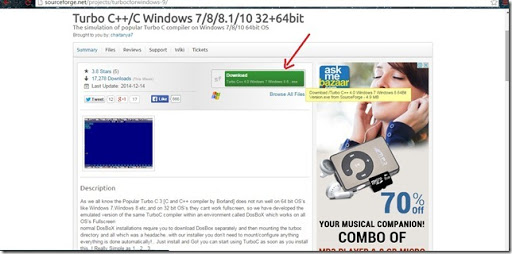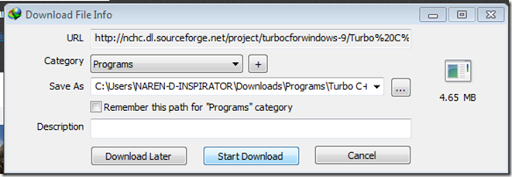Then Install the Turbo c++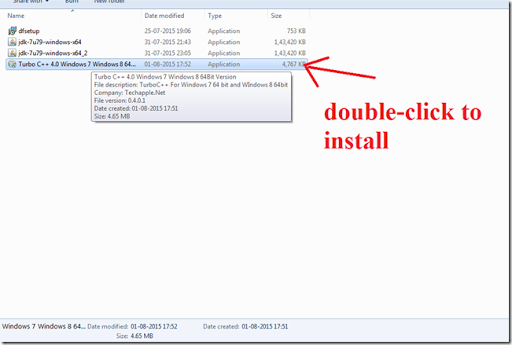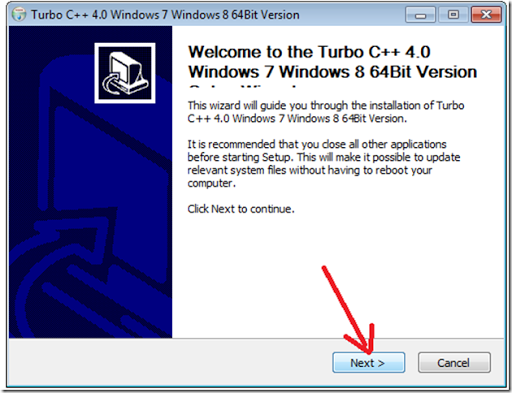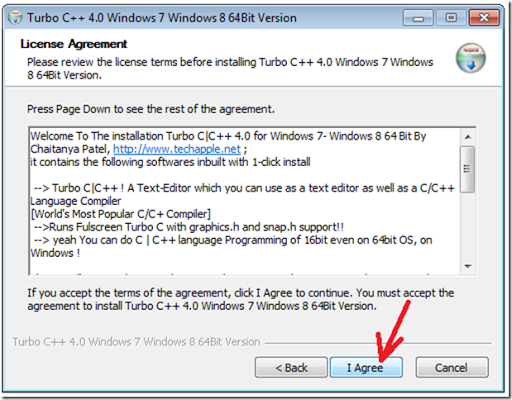Click The Install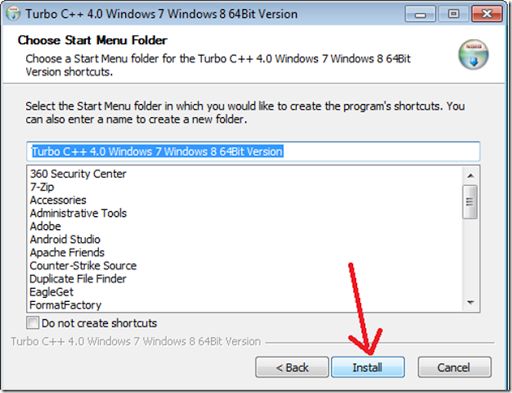Then Installing will be started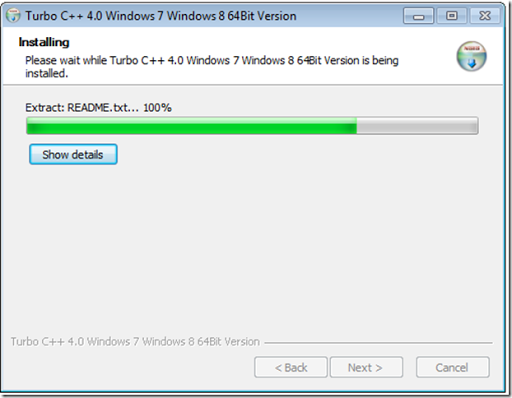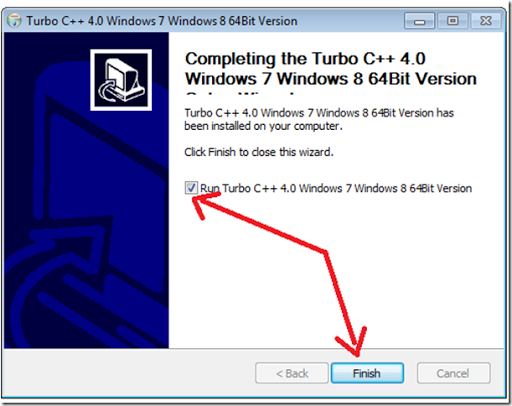After the installation completed the Turbo c++ compiler will be opened with a pre-defined graphic program.
[Note: press ALT+Enter to make the compiler minimize and maximize.]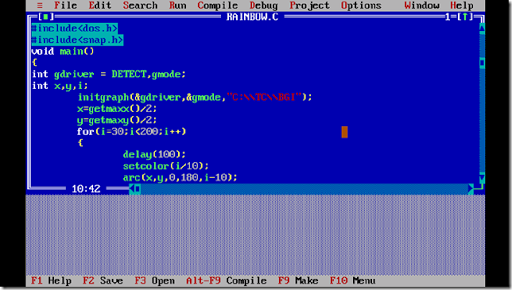Then go to FILE click new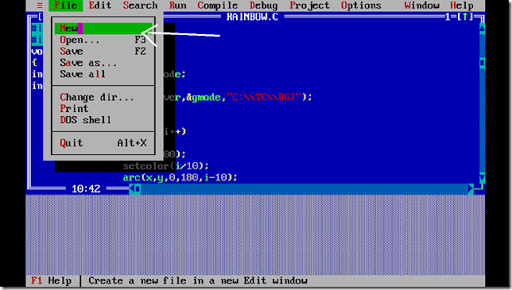So after the new screen is opened save it as filename.cpp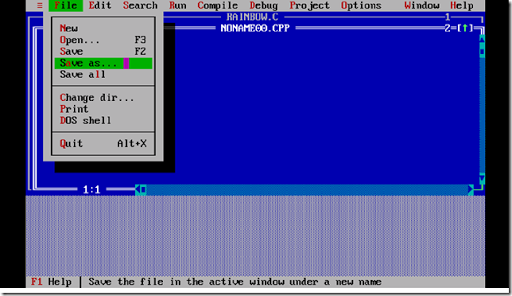For example demo.cpp and click OK

SO type the following simple C++ program to print “This is my first c++ program” in the output screen
#include<iostream.h>
#include<conio.h>
void main()
{
clrscr();
cout<<“this is my first c program”;
getch();
}

After typing the program save it again,  we have to compile the program, to compile click the Compile option or you can use the short cut key  Alt+F9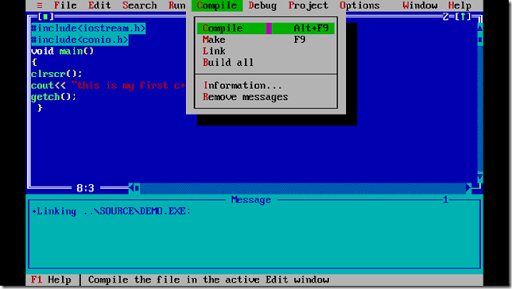Then it checks for any error and it will display the number of errors and warnings. If the program is right it will show the error and warnings as 0.
click the Run option or use the shortcut key Ctrl+F9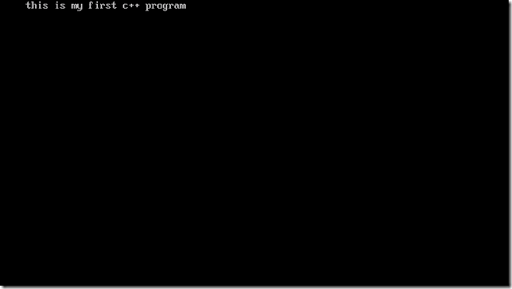we have successfully got the output.
Thus the simple program in C++ is implemented successfully.
In the upcoming tutorials I will teach C++ and the Object Oriented Concepts  more specifically…